# Class 9 RD Sharma Solutions – Chapter 13 Linear Equation in Two Variable- Exercise 13.4

### Question 1: Give the geometric representations of the following equations(a) on the number line (b) on the Cartesian plane:(i) x = 2 (ii) y + 3 = 0 (iii) y = 3 (iv) 2x + 9 = 0 (v) 3x – 5 = 0

Solution:

(i) x = 2
The representation of equation on the number line: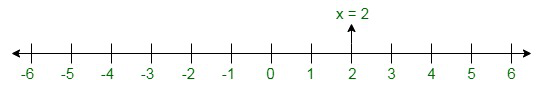The representation of equation on the Cartesian plane: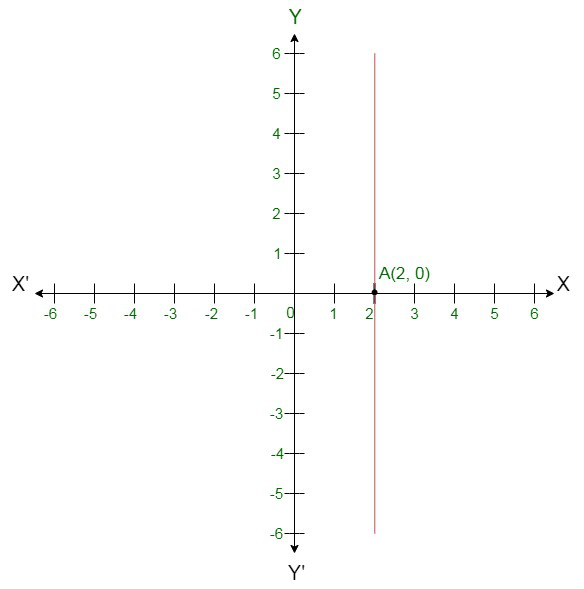(ii) y + 3 = 0

or y = -3
The representation of the equation on the number line: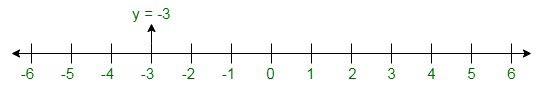The representation of the equation on the Cartesian plane: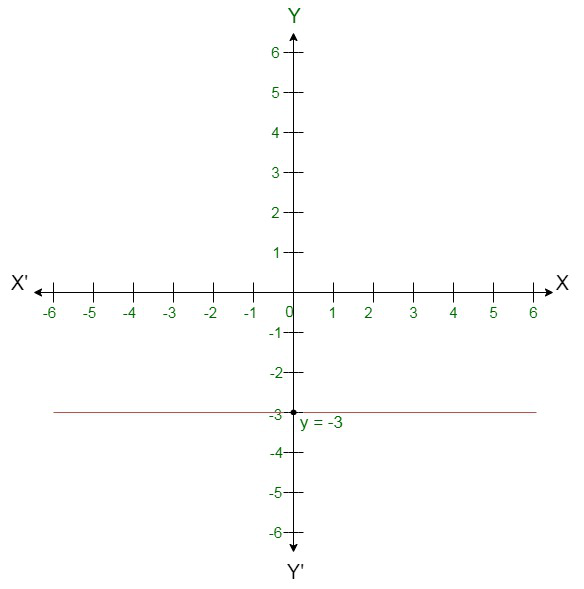(iii) y = 3
The representation of equation on the number line: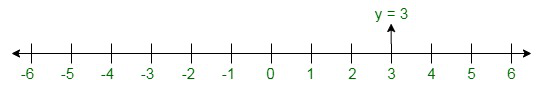The representation of equation on the Cartesian plane: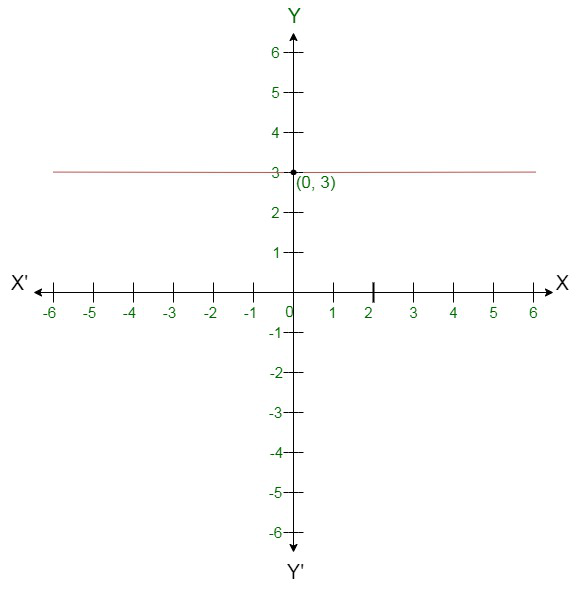(iv) 2x + 9 = 0
or x =  -9
2
The representation of equation on the number line: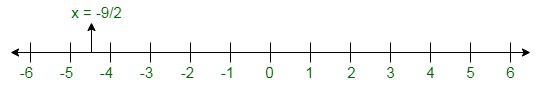The representation of equation on the Cartesian plane: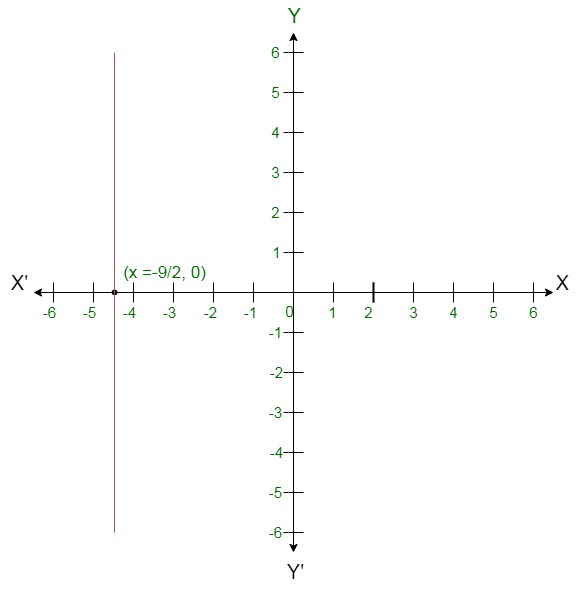(v) 3x – 5 = 0
or x =
3
The representation of equation on the number line: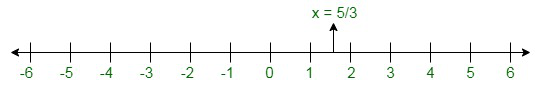The representation of equation on the Cartesian plane: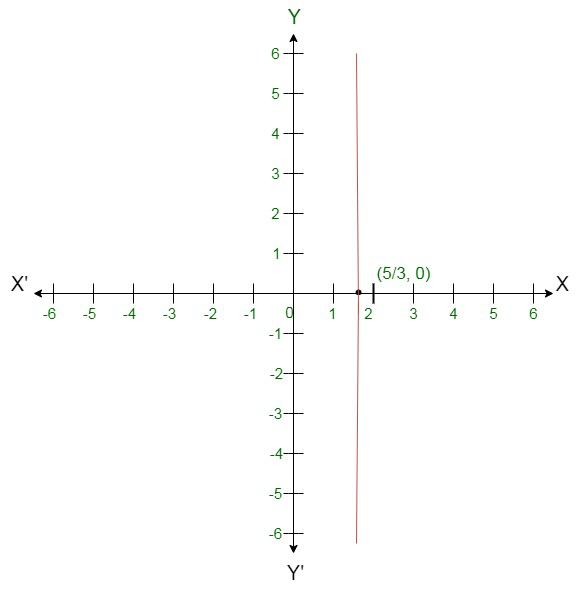### Question 2: Give the geometrical representation of 2x + 13 = 0 as an equation in(i) one variable (ii) two variables

Solution:

2x + 13 = 0
(i) Isolate given equation in x
Subtract 13 from both the sides
2x + 13 – 13 = 0 – 13
2x = -13
Divide each side by 2
x =  – 13  = -6.5
2
Which is an equation in one variable.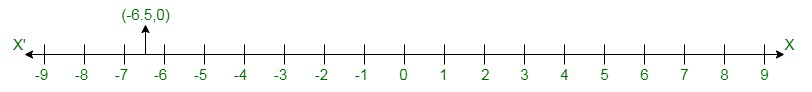(ii) 2x + 13 = 0 can be written as 2x + 0y + 13 = 0
The representation of the solution on the Cartesian plane: A line parallel to y axis passing through the point (-13  , 0):
2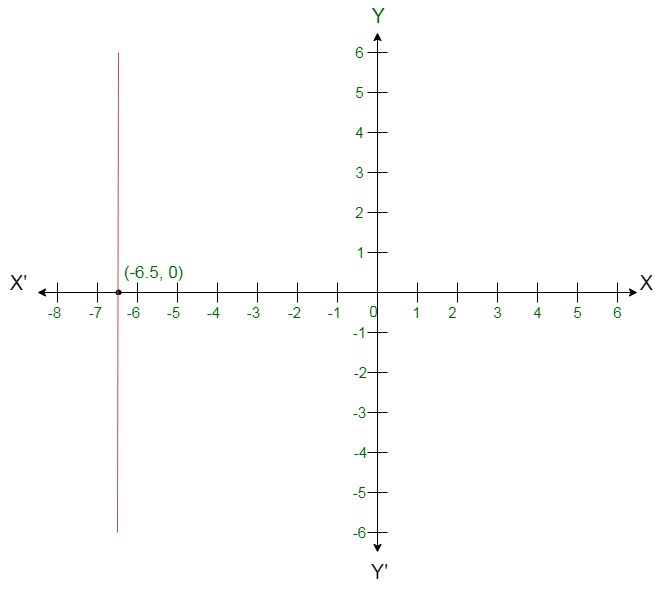### Question 3: Write the equation of a line passing through the point (0, 4) and parallel to x-axis.

Solution

Here, x-coordinate is 0 and y-coordinate is 4, so equation of the line passing through the point (0, 4) is y = 4.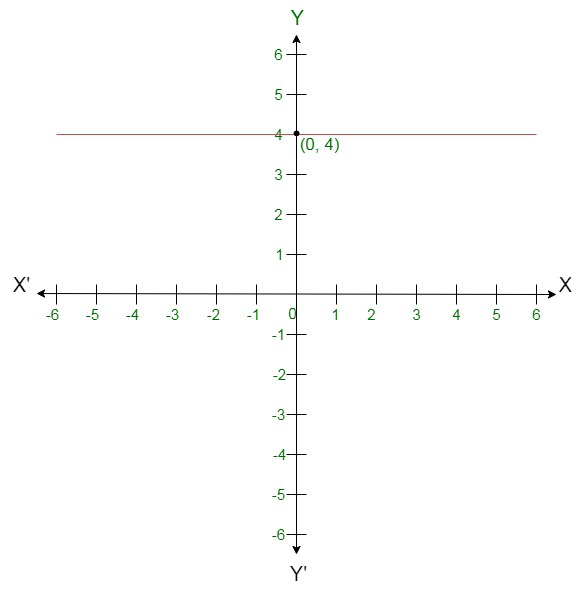### Question 4: Write the equation of a line passing through the point (3, 5) and parallel to x-axis.

Solution

Here x-coordinate = 3 and y-coordinate = 5
Since required line is parallel to x-axis, so equation of line is y = 5.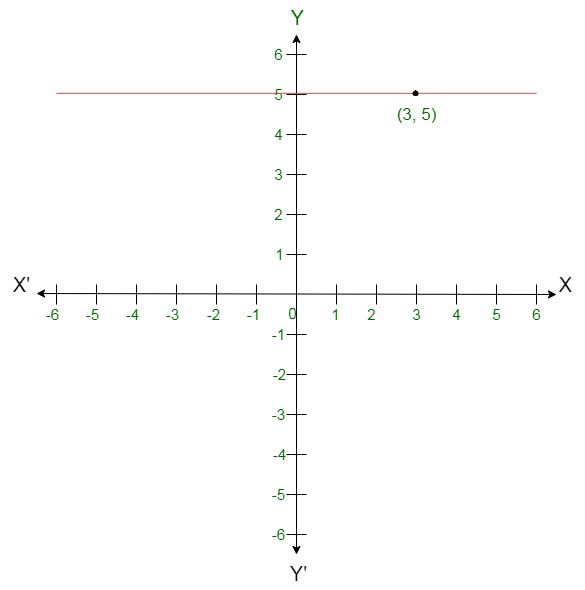### Question 5: Write the equation of a line parallel to y-axis and passing through the point (-3, -7)

Solution:

Here x-coordinate = -3 and y-coordinate = -7
Since required line is parallel to y-axis, so equation of line is x = -3.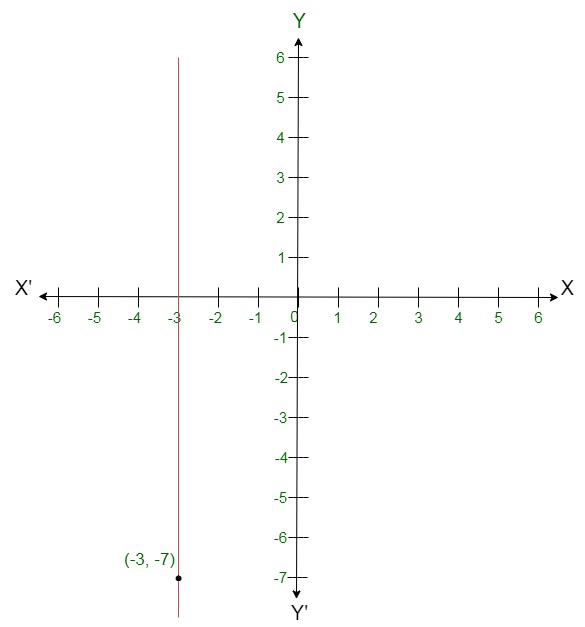Whether you're preparing for your first job interview or aiming to upskill in this ever-evolving tech landscape, GeeksforGeeks Courses are your key to success. We provide top-quality content at affordable prices, all geared towards accelerating your growth in a time-bound manner. Join the millions we've already empowered, and we're here to do the same for you. Don't miss out - check it out now!

Previous
Next